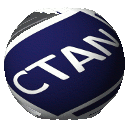# The `mathtools` bundle

`mathtools`

The `mathtools` package provides many useful tools for mathematical typesetting. It is based on `amsmath` and fixes various deficiencies of `amsmath` and standard . It provides:

• Extensible symbols, such as brackets, arrows, harpoons, etc.
• Various symbols such as `\coloneqq` (:=).
• Easy creation of new tag forms.
• Showing only the referenced equations.
• Extensible arrows, harpoons and hook arrows.
• Starred versions of the `amsmath` matrix environments for specifying the column alignment.
• More building blocks: multlined, cases-like environments, new gathered environments.
• Math versions of `\makebox`, `\llap`, `\rlap`, etc.
• Cramped math styles.
• and more ...

`mathtools` requires `mhsetup`.

`empheq`

The `empheq` package is a visual markup extension designed to function on top of `amsmath`. It features:

• Boxing multi line math displays while leaving equation numbers untouched at the margin. Any kind of box will do.
• Making the `ntheorem` package place end-of-theorem markers perfectly.
• Placing arbitrary material on either side of math displays. This includes delimiters that automatically scale to the correct size.

`empheq` requires `mathtools`.

`mhsetup`

The `mhsetup` package defines various programming tools needed by both `empheq` and `mathtools`. In the future, most of these tools will probably be an integral part of 3.

## mathtools – Mathematical tools to use with amsmath

Mathtools provides a series of packages designed to enhance the appearance of documents containing a lot of mathematics. The main backbone is amsmath, so those unfamiliar with this required part of the system will probably not find the packages very useful.

Mathtools provides many useful tools for mathematical typesetting. It is based on amsmath and fixes various deficiencies of amsmath and standard . It provides:

• Extensible symbols, such as brackets, arrows, harpoons, etc.;
• Various symbols such as \coloneqq (:=);
• Easy creation of new tag forms;
• Showing equation numbers only for referenced equations;
• Extensible arrows, harpoons and hookarrows;
• Starred versions of the amsmath matrix environments for specifying the column alignment;
• More building blocks: multlined, cases-like environments, new gathered environments;
• Maths versions of \makebox, \llap, \rlap etc.;
• Cramped math styles; and more...

Mathtools requires mhsetup.

 Package mathtools Repository https://github.com/latex3/mathtools Version 1.29 2022-06-29 Licenses The LaTeX Project Public License 1.3c Copyright 2002–2011 Morten Høgholm2012–2019 Lars Madsen2020–2022 Lars Madsen, The LaTeX3 Project Maintainer The LaTeX Project TeamLars MadsenMorten Høgholm (inactive)Will Robertson (inactive)Joseph Wright (inactive) TDS archive `mathtools.tds.zip` Contained in TeX Live as mathtoolsMiKTeX as mathtools Topics Maths See also mhsetupempheq